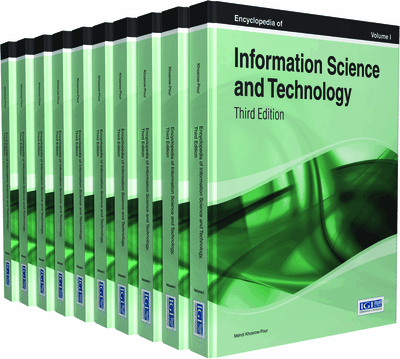# Consensus Clustering

Sławomir T. Wierzchoń (Institute of Computer Science, Polish Academy of Sciences, Poland)
DOI: 10.4018/978-1-4666-5888-2.ch162

Top

## Introduction

Clustering is an exploratory activity relying upon dividing a given collection X of objects, or entities, into a set of categories, called groups or clusters. Consensus clustering has been proposed to overcome some drawbacks of individual clustering algorithms. Usually we assume that the clusters are disjoint subsets of X such that the objects belonging to a single cluster are sufficiently similar to each other (i.e. the clusters should be homogeneous), while objects from different clusters should be sufficiently diversified (i.e. clusters should be well separated). Splitting given collection into disjoint clusters is termed hard clustering. Otherwise we say about soft clustering, i.e. – depending on the formalism used – probabilistic or fuzzy clustering. An unacquainted reader is referred to the chapter “Minimum sum-of-squares clustering’’ for details.

The most popular clustering algorithm is the k-means algorithm producing hard partitions – consult (Jain, 2010) for historical background and deeper discussion of its current improvements and variations. Soft version of the algorithm, called fuzzy c-means, was proposed by Bezdek (1981). This author used c to name the number of clusters, hence the name of the algorithm. Both the algorithms minimize the squared-error criteria, they are computationally efficient and do not require the user to specify many parameters. However, there are three main disadvantages of both the algorithms. First, they require that the entities must be represented as points in n-dimensional Euclidean space. To alleviate this assumption Hathaway, Davenport and Bezdek (1989) introduced relational version of fuzzy c-means algorithm: instead of the distance between the points representing the objects, a similarity measure between all pair of objects was used. In case of hard partitions the k-medoids algorithm was proposed: here a dissimilarity measure between pairs of objects replaces the distance measure – see e.g. section 14.3.10 in (Hastie, Tibshirani, & Friedman, 2009). The second disadvantage results from the way in which objects are assigned to the clusters. Namely, in case of hard k-means each object is located to the cluster with nearest centroid (empirical mean of the cluster). Thus resulting clusters are spherical (more precisely, they are Voronoi regions). A similar assignment rule is used in the fuzzy c-means algorithm. Third disadvantage is that, the clusters should be of approximately similar cardinality and of similar shape. In case of unbalanced clusters erroneous results are frequently obtained. Similarly, if one cluster is located within a ball of small radius and the second – within an ellipsoid with one axis much greater than others, we can obtain erroneous results. Examples of “easy” and “difficult” data are depicted in Figure 1. Left panel presents compact, well separated, convex and linearly separated clusters, while non-convex clusters that are not linearly separated are shown in right panel.

To avoid these disadvantages, the ideas of ensemble methods used by machine learning community to improve results of classification methods, have been adapted to the requirements of clustering. In general, the ensemble methods use multiple models to obtain better predictive performance than could be obtained from any of the constituent models. When transposed in the field of unsupervised learning (i.e. clustering) this idea translates to generating multiple partitions of the same data. By combining these partitions, it is possible to obtain a good data partition even when the clusters are not compact and/or well separated (Jain, 2010). Consensus clustering seems to be especially recommendable to analyze huge datasets. As noted in (Hore, Hall, & Goldgof, 2009): “The advantage of these approaches is that they provide a final partition of data that is comparable to the best existing approaches, yet scale to extremely large datasets. They can be 100,000 times faster while using much less memory.”

## Key Terms in this Chapter

Unsupervised Learning: Contrary to the supervised learning we have only the data set X = { x 1 , …, x m } of m objects or entities. The aim of unsupervised learning is to find inner structure in this set, or equivalently, to find some regularities in this set. Clustering is a possible tool used to extract such information, but other approaches (e.g. self organizing maps) may be used as well.

NP-Hard Problems: Very hard computational problems. The shorthand NP means “non-deterministic polynomial-time.”

Voronoi Regions: See chapter “Minimum sum-of-squares clustering.”

Supervised Learning: The problem of supervised learning is formulated as follows: Let X = { x 1 , …, x m } be a set of m objects or entities. Suppose these objects come from k classes and the membership to each object to exactly one class is known in advance. Then such a set is termed a training set T . It has the form T = {( x 1 , l 1 ) …, ( x m , l m )} where l i denotes the label of a class to which i -th object belongs. The aim of supervised learning is to find a mapping f : X ? L , such that f ( x i ) = l i , for all i = 1, …, m . The function f is said to be decision function or decision rule. If the labels l i are not known we say about unsupervised learning. Its task is to discover a structure in the set X .

Meta-Heuristics: In computer methods used in optimization, by metaheuristic we understand an algorithm that finds a satisfactory solution to a given optimization problem by iteratively trying to improve a candidate solution with respect to a given measure of quality. When a single solution is iteratively improved we say about local search algorithm, and when a set of candidate solutions is simultaneously modified, we say about population-based search methods. A well known example of such methods is evolutionary algorithm, being elaborated version of so-called genetic algorithm.

## Complete Chapter List

Search this Book:
Reset